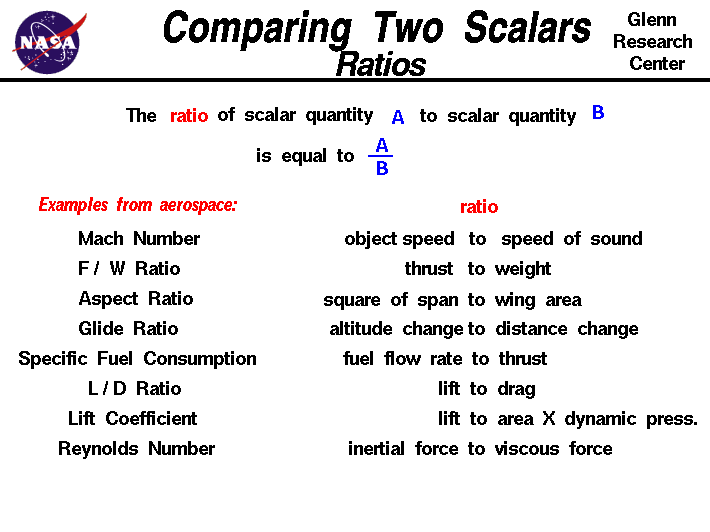+ Text Only Site
+ Non-Flash Version
+ Contact GlennMath and science were invented by humans to describe and understand the world around us. We observe that there are some quantities and processes in our world that depend on the direction in which they occur, and there are some quantities that do not depend on direction. For example, the volume that an object occupies in space does not depend on its direction. If we have a 5 cubic foot block of iron and we move it up and down and then left and right, we still have a 5 cubic foot block of iron. On the other hand, the location, of an object does depend on direction. If we move the 5 cubic foot block 5 miles to the north, the resulting location is very different than if we moved it 5 miles to the east. Mathematicians and scientists call a quantity which depends on direction a vector quantity and a quantity which does not depend on direction is called a scalar quantity. Vector quantities have two characteristics, a magnitude and a direction; scalar quantities have only a magnitude. To better understand our world, engineers often compare scalar quantities by using the ratio of the magnitude of the scalars. Comparing two vectors is more complex because you have to consider both magnitude and direction. The ratio of a scalar quantity a to a scalar quantity b is equal to a divided by b. ratio = a / b Here are some simple rules for working with ratios that apply to all scalar quantities: If the value of the ratio is 1.0, then a = b . If the value of the ratio is greater than 1.0, then a > b. If the value of the ratio is less than 1.0, then a < b . If the value of the ratio is nearly zero, then a is very much less than b or a is nearly zero. If the value of the ratio is very large, then a is very much larger than b or b is nearly zero. Here are some examples of ratios that are used in aerospace. The Mach number is the ratio of the speed of an object to the speed of sound in a gas. The Mach number defines the various flight regimes of an aircraft and the importance of compressibility of the flow. The thrust to weight ratio of a rocket determines the g-loading on the payload and structure. The specific fuel consumption (sfc) for jet engines is the ratio of the fuel flow rate to the amount of thrust produced. An efficient engine has a low value of sfc and burns little fuel to produce thrust. A similar parameter for rocket engines is the specific impulse (Isp). The Isp is the ratio of the thrust to the weight flow of the propellants burned. In this case, a rocket with a high Isp is better than a low Isp because it produces more thrust for equal propellant weight flow. The specific heat ratio is the ratio of the specific heat at constant pressure to the specific heat at constant volume. It is a property of a gas which appears in many compressible flow equations. The value for air at standard conditions is 1.4. The drag coefficient is a number which relates the aerodynamic forces on an object to the dynamic pressure times the area of the object. The Reynolds number is the ratio of the inertia forces in a fluid to the viscous forces. This ratio appears in boundary layer theory and multiplies the viscous terms of the Navier-Stokes Equations of fluid dynamics. This is only a partial list of the many ratios which engineers use every day. he engine fuel/air ratio and compression ratio help to determine engine performance. Activities: Guided Tours Navigation ..Beginner's Guide Home Page+ Inspector General Hotline + Equal Employment Opportunity Data Posted Pursuant to the No Fear Act + Budgets, Strategic Plans and Accountability Reports + Freedom of Information Act + The President's Management Agenda + NASA Privacy Statement, Disclaimer, and Accessibility CertificationEditor: Tom Benson NASA Official: Tom Benson Last Updated: Jun 12 2014 + Contact Glenn